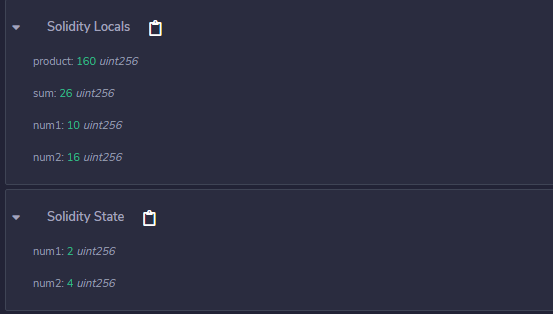# Solidity – View and Pure Functions

• Last Updated : 11 May, 2022

The view functions are read-only function, which ensures that state variables cannot be modified after calling them. If the statements which modify state variables, emitting events, creating other contracts, using selfdestruct method, transferring ethers via calls, Calling a function which is not ‘view or pure’, using low-level calls, etc are present in view functions then the compiler throw a warning in such cases. By default, a get method is view function.

Example: In the below example, the contract Test defines a view function to calculate the product and sum of two unsigned integers.

## Solidity

 `// Solidity program to  ` `// demonstrate view ` `// functions ` `pragma solidity ^0.5.0; ` ` `  `// Defining a contract ` `contract` `Test { ` `     `  `    ``// Declaring state  ` `    ``// variables ` `    ``uint num1 = 2;  ` `    ``uint num2 = 4; ` ` `  `   ``// Defining view function to   ` `   ``// calculate product and sum  ` `   ``// of 2 numbers ` `   ``function getResult( ` `   ``) ``public` `view returns( ` `     ``uint product, uint sum){ ` `       ``uint num1 = 10; ` `       ``uint num2 = 16; ` `      ``product = num1 * num2; ` `      ``sum = num1 + num2;  ` `   ``} ` `}`

Output :The pure functions do not read or modify the state variables, which returns the values only using the parameters passed to the function or local variables present in it. If the statements which read the state variables, access the address or balance, accessing any global variable block or msg, calling a function which is not pure, etc are present in pure functions then the compiler throws a warning in such cases.

Example: In the below example, the contract Test defines a pure function to calculate the product and sum of two numbers.

## Solidity

 `// Solidity program to   ` `// demonstrate pure functions ` `pragma solidity ^0.5.0; ` ` `  `// Defining a contract ` `contract` `Test { ` ` `  `    ``// Defining pure function to  ` `    ``// calculate product and sum ` `   ``// of 2 numbers ` `   ``function getResult( ` `   ``) ``public` `pure returns( ` `     ``uint product, uint sum){ ` `      ``uint num1 = 2;  ` `      ``uint num2 = 4; ` `      ``product = num1 * num2; ` `      ``sum = num1 + num2;  ` `   ``} ` `} `

Output :My Personal Notes arrow_drop_up
Recommended Articles
Page :